Search IntMath
Close

# IntMath Newsletter: Unit circle, gmail, graphs

By Murray Bourne, 06 Oct 2010

6 Oct 2010

1. Math tip: unit circles
2. Why do people use gmail most on Saturdays?
3. Math tip: Implicit functions (I heart math)
4. Resource: Free math graph software
5. Friday math movie: Mandelbox Trip
6. Final thought - enthusiasm

## 1. Unit Circle: an Introduction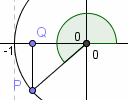The unit circle ties together 3 great strands in mathematics: Euclidean geometry, coordinate geometry and trigonometry. Read more: Unit Circle: an Introduction

## 2. Saturday is gmail day, Monday is not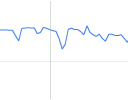Why do people access Google maps least on a Sunday? And why is gmail traffic so periodic? Read more: Saturday is gmail day, Monday is not

## 3. I heart math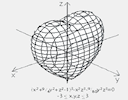We learn about implicit functions in 2-D and 3-D and how they give us familiar shapes. Read more: I heart math

## 4. Free math graphing software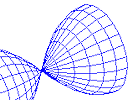Here are 2 more free math graphing utilities. Free math graphing software

## 5. Friday math movie: Mandelbox trip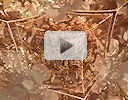Here's an interesting trip through a 3-D mathematical art space, based on fractals. Read more: Friday math movie: Mandelbox trip

## 6. Final thought – Enthusiasm

Many people struggle with math. Entrepreneur Walter Chrysler, founder of the Chrysler auto company, famously said:

The real secret of success is enthusiasm.

Until next time, enjoy whatever you learn.

### Comment Preview

HTML: You can use simple tags like <b>, <a href="...">, etc.

To enter math, you can can either:

1. Use simple calculator-like input in the following format (surround your math in backticks, or qq on tablet or phone):
a^2 = sqrt(b^2 + c^2)
(See more on ASCIIMath syntax); or
2. Use simple LaTeX in the following format. Surround your math with $$ and $$.
$$\int g dx = \sqrt{\frac{a}{b}}$$
(This is standard simple LaTeX.)

NOTE: You can mix both types of math entry in your comment.